# Gaussian Elimination

• Introduction

• Resolution Method: Gaussian Elimination and the Rouché-Capelli theorem.

• 10 Resolved Systems by Gaussian Elimination

## Introduction

A system of equations (linear) is a group of (linear) equations with various unknown factors. Generally speaking, the unknown factors appear in various equations.

What an equation with various unknown factors does is relates them amongst each other.

Solving a system consists in finding the value for the unknown factors in a way that verifies all the equations that make up the system.

• If there is a single solution (one value for each unknown factor) we will say that the system is Consistent Independent System (CIS).

• If there are various solutions (the system has infinitely many solutions), we say that the system is a Consistent Dependent System (CDS). .

• If there is no solution, and this will happen if there are two or more equations that can't be verified at the same time, we say it's an Inconsistent System (IS). For example, the following system of equations

$$\begin{cases} \begin{array}{lcl} y & = & 0 \\ 2x + y & = & 0 \\ 2x + y & = & 2 \end{array} \end{cases}$$

is inconsistent because of we obtain the solution x = 0 from the second equation and, from the third, x = 1.

In this section we are going to solve systems using the Gaussian Elimination method, which consists in simply doing elemental operations in row or column of the augmented matrix to obtain its echelon form or its reduced echelon form (Gauss-Jordan).

## Resolution Method

1. We apply the Gauss-Jordan Elimination method: we obtain the reduced row echelon form from the augmented matrix of the equation system by performing elemental operations in rows (or columns).

2. Once we have the matrix, we apply the Rouché-Capelli theorem to determine the type of system and to obtain the solution(s), that are as:

Let A·X = B be a system of m linear equations with n unknown factors, m and n being natural numbers (not zero):

• AX = B is consistent if, and only if,

$$rank(A)=rank(A|B)$$

• AX = B is consistent independent if, and only if,

$$rank(A)=n=rank(A|B)$$

Note: The elemental operations in rows or columns allow us to obtain equivalent systems to the initial one, but with a form that simplifies obtaining the solutions (if there are). Also, there are quicker tools to work out the solutions in the CIS, like Cramer's rule.

# Solved Systems

### System 1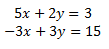Show Solution

### System 2Show Solution

### System 3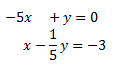Show Solution

### System 4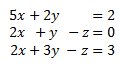Show Solution

### System 5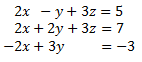Show Solution

### System 6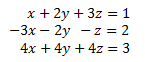Show Solution

### System 7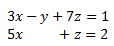Show Solution

### System 8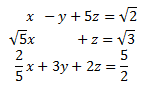Show Solution

### System 9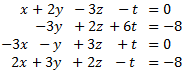Show Solution

### System 10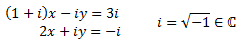Show Solution